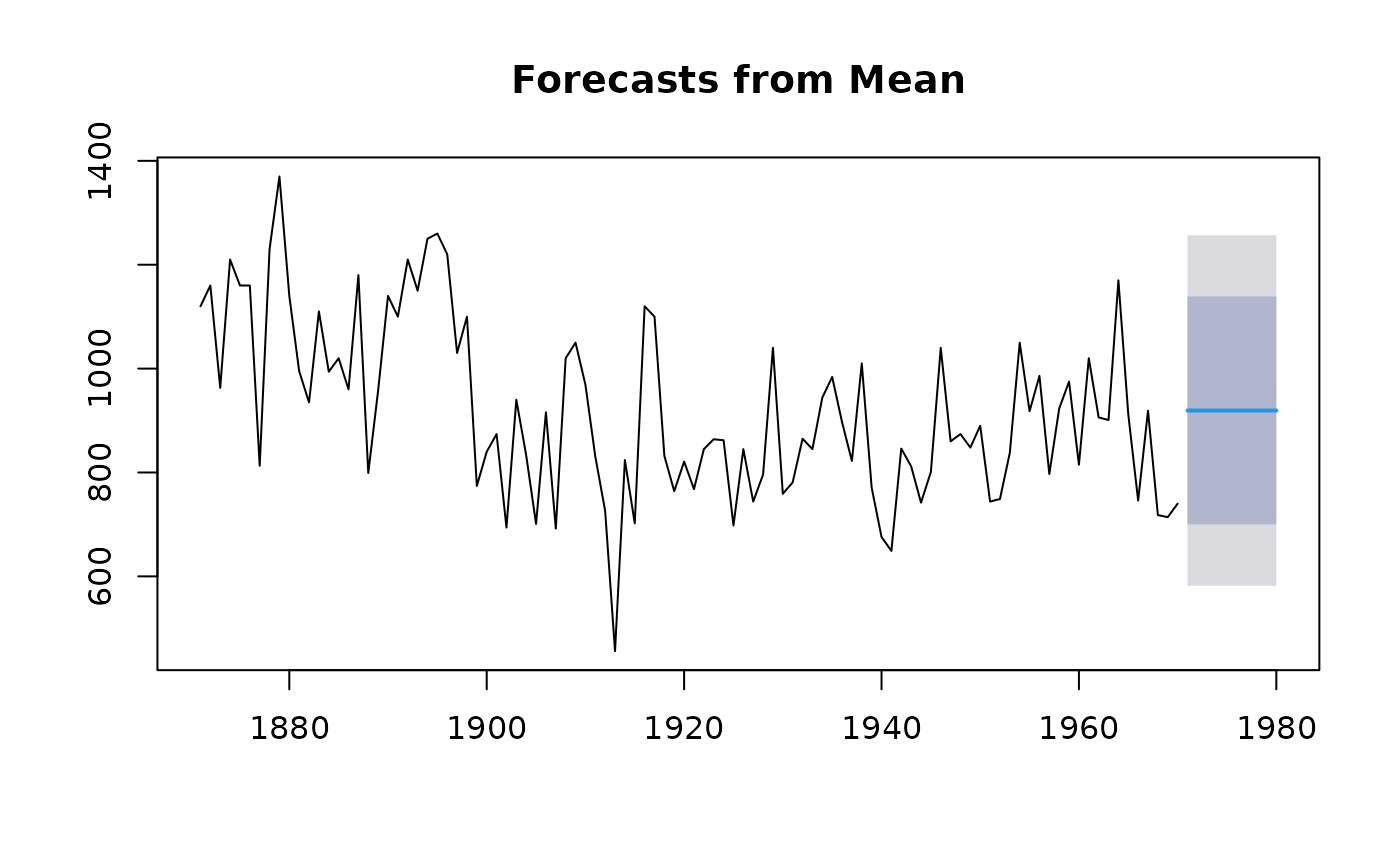Returns forecasts and prediction intervals for an iid model applied to y.

meanf(
y,
h = 10,
level = c(80, 95),
fan = FALSE,
lambda = NULL,
bootstrap = FALSE,
npaths = 5000,
x = y
)

## Arguments

y a numeric vector or time series of class ts Number of periods for forecasting Confidence levels for prediction intervals. If TRUE, level is set to seq(51,99,by=3). This is suitable for fan plots. Box-Cox transformation parameter. If lambda="auto", then a transformation is automatically selected using BoxCox.lambda. The transformation is ignored if NULL. Otherwise, data transformed before model is estimated. Use adjusted back-transformed mean for Box-Cox transformations. If transformed data is used to produce forecasts and fitted values, a regular back transformation will result in median forecasts. If biasadj is TRUE, an adjustment will be made to produce mean forecasts and fitted values. If TRUE, use a bootstrap method to compute prediction intervals. Otherwise, assume a normal distribution. Number of bootstrapped sample paths to use if bootstrap==TRUE. Deprecated. Included for backwards compatibility.

## Value

An object of class "forecast".

The function summary is used to obtain and print a summary of the results, while the function plot produces a plot of the forecasts and prediction intervals.

The generic accessor functions fitted.values and residuals extract useful features of the value returned by meanf.

An object of class "forecast" is a list containing at least the following elements:

model

A list containing information about the fitted model

method

The name of the forecasting method as a character string

mean

Point forecasts as a time series

lower

Lower limits for prediction intervals

upper

Upper limits for prediction intervals

level

The confidence values associated with the prediction intervals

x

The original time series (either object itself or the time series used to create the model stored as object).

residuals

Residuals from the fitted model. That is x minus fitted values.

fitted

Fitted values (one-step forecasts)

## Details

The iid model is $$Y_t=\mu + Z_t$$ where $$Z_t$$ is a normal iid error. Forecasts are given by $$Y_n(h)=\mu$$ where $$\mu$$ is estimated by the sample mean.

rwf
nile.fcast <- meanf(Nile, h=10)